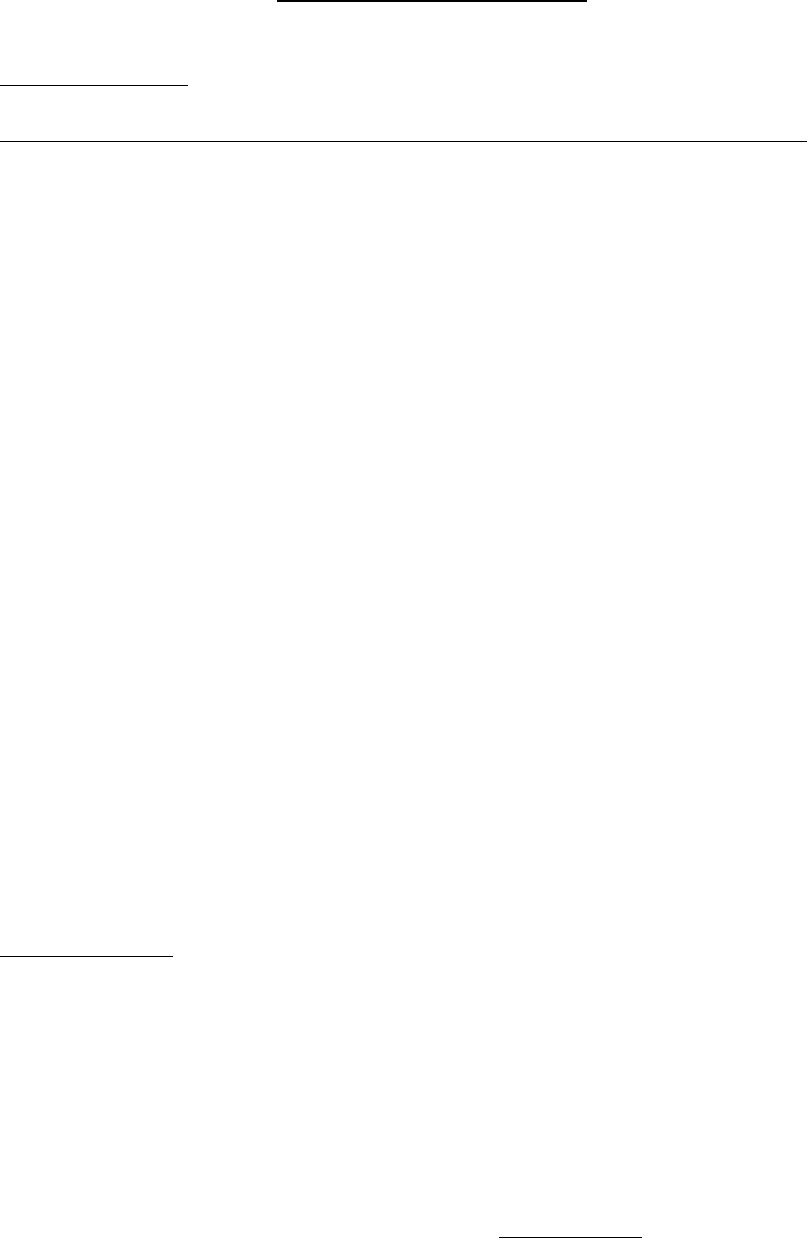# COMMERCE 2AB3 Lecture Notes - Lecture 10: Variable Cost, Fixed Cost, Cost Driver

25 views3 pages
School
DepartmentCHAPTER 10 – CLASS NOTES
Cost Estimation or Regression Analysis For Cost Prediction
January 13, 2016
Written Problem On Exam #1 (10 Marks): PREDICTING COST BEHAVIOUR
1. Production of Trucks
2. Union Gas
Minimum Amount: \$21
0 – 50 m3 = 0.04 c/m3
51 – 100 m3 = 0.06 c/m3
Free thereafter: you’re not paying for more than the maximum \$.
Cost Estimation
2 Assumptions:
1. All costs are linear.
2. Only 1 cost driver explains cost behavior.
y = a + (b) (x) COST DRIVER
T.F.C U.V.C
ONE: HIGH-LOW METHOD OF COST ESTIMATION:
Take the highest & the lowest observation of the COST DRIVER.
PRACTISE QUESTION - EXAMPLE 1: WRITE ON NOTES CH10
find more resources at oneclass.com
find more resources at oneclass.com
Unlock document

This preview shows page 1 of the document.
Unlock all 3 pages and 3 million more documents.

## Document Summary

Cost estimation or regression analysis for cost prediction. Written problem on exam #1 (10 marks): predicting cost behaviour: production of trucks, union gas. Free thereafter: you"re not paying for more than the maximum \$. 2 assumptions: all costs are linear, only 1 cost driver explains cost behavior. y = a + (b) (x) cost driver. Take the highest & the lowest observation of the cost driver. Practise question - example 1: write on notes ch10. We don"t care about the highest or lowest cost, we care about the highest and lowest cost driver (x). (so look at total operating hours). Step 1: slope = gradient y=rise/run= change in y/change in x. 23030-22510 / 3614 3325 = 1. 80 = b. Step 2: use either the highest or lowest data point to calculate a (fixed cost). Using the highest point: 23030 = a + 1. 8(3,614) Step 3: cost equation (tc) total cost = 16,525 + . 80.

## Get access

\$8 USD/m\$10 USD/m
Billed \$96 USD annuallyHomework Help
Study Guides
Textbook Solutions
Class Notes
Textbook Notes
Booster Class